# Civil Engineering - Theory of Structures

Exercise : Theory of Structures - Section 2
46.
The area of the core of a column of cross sectional area A, is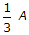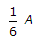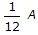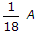Explanation:
No answer description is available. Let's discuss.

47.
A simply supported beam carries a varying load from zero at one end and w at the other end. If the length of the beam is a, the shear force will be zero at a distance x from least loaded point where x is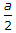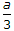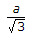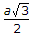Explanation:
No answer description is available. Let's discuss.

48.
The locus of reaction of a two hinged semi-circular arch, is
straight line
parabola
circle
hyperbola.
Explanation:
No answer description is available. Let's discuss.

49.
The ratio of the area of cross-section of a circular section to the area of its core, is
4
8
12
16